# Chaos Theory

11 Jan 2010
Progress: Completed

I find chaos theory fascinating, the idea that something can be both deterministic and unpredictable. What better way is there to investigate than a computer model – one with no random parameters, and input values known to a ridiculous number of decimal places. I wrote this program and write-up for an assignment in uni. Needless to say I got the highest mark possible.

Predictability of a Double Pendulum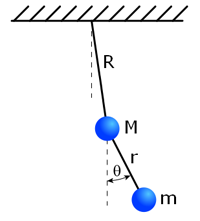Figure 1
Double pendulum diagram
showing M, m, R, r, and θ

A simulation of a double pendulum was constructed to investigate the predictability of its final position after a set amount of time, for different initial conditions. Certain ranges were found to be predictable while others were chaotic. The boundaries of these regions showed a direct correlation to where the lower pendulum inverted.

Many natural systems exhibit chaos, such as the weather. Despite their deterministic nature these systems can be unpredictable due to their extreme sensitivity to initial conditions. Knowing what defines the chaotic regions is important in many fields.

In the simplest (Ref1) double pendulum (Figure1) m << M, thus the motion (amplitude) of M can be approximated by (eq.1).

(eq.1)where a0 and τ are constants of amplitude and period of M. Hence m is modelled as a single pendulum under acceleration a (eq.2&3)

(eq.2&3)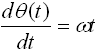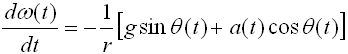where ω is the angular velocity of m. Constants r, g are arbitrarily chosen to be 1. Initial values of ω, θ are chosen to be 0.0 and 0.1, respectively.

The first simulation evolves for tmax=100 seconds and records the θfinal. This simulation is repeated through a range of values for a0 and τ, and plotted to a graph (Figure2). Each data-point is a shade of gray corresponding to the value of theta; it is not the brightness that is of relevance but more how it relates to adjacent points. Thus areas forming a smooth gradient represent predictable regions, while the seemingly stochastic areas correspond to chaos. Figure3 shows the same area with tmax=400 seconds. The boundary between chaos and predictability is clearer.Figure 2.
Values of θ for 0.21 < a0 < 0.25 and 7 < τ < 9 with tmax = 100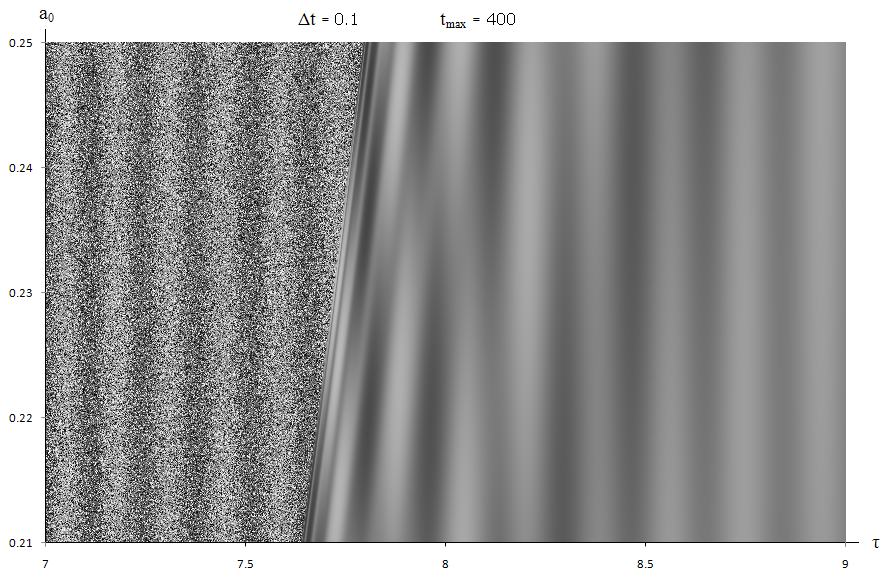Figure 3.
Values of θ for 0.21 < a0 < 0.25 and 7 < τ < 9 with tmax = 400

A phase-space plot (ω verses θ) for a single pendulum would be a circle. Figures 4&5 show phase-space plots for simulations inside and outside the chaotic boundary. The chaotic pendulum’s plot stretching to the left demonstrates θ leaving its normal range, i.e. it made a full rotation. Investigating this as the reason for the chaotic region, the program was modified to identify regions where the lower pendulum inverted. This was achieved by measuring the proportion of time θ escaped the range (-π,π) and highlighting the graph in red to represent it. (Figures6&7)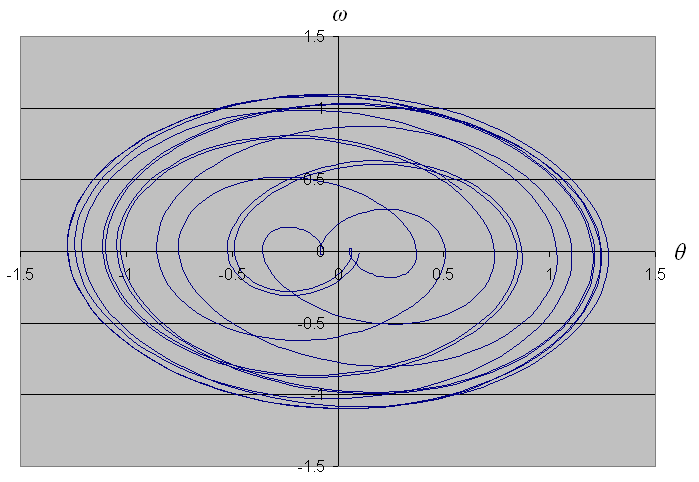Figure 4.
Phase-space plot with a0 = 0.251, τ = 8 and tmax = 100 The pendulum swings normally, with just a few wobbles.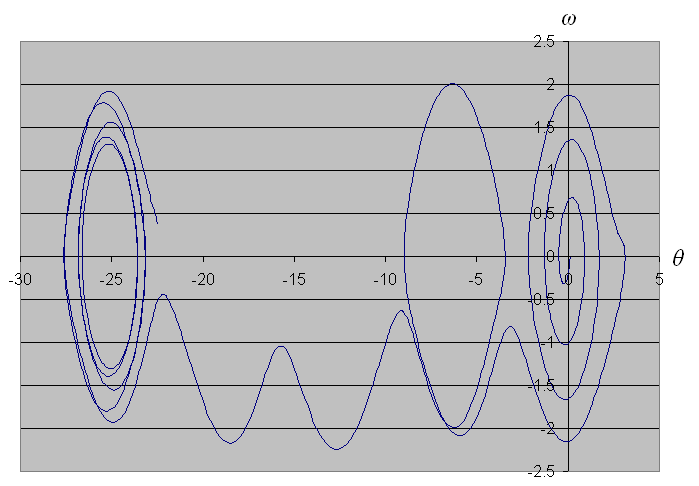Figure 5.
Phase-space plot with a0 = 0.251, τ = 7 and  tmax = 100 The pendulum has clearly done several full inversions.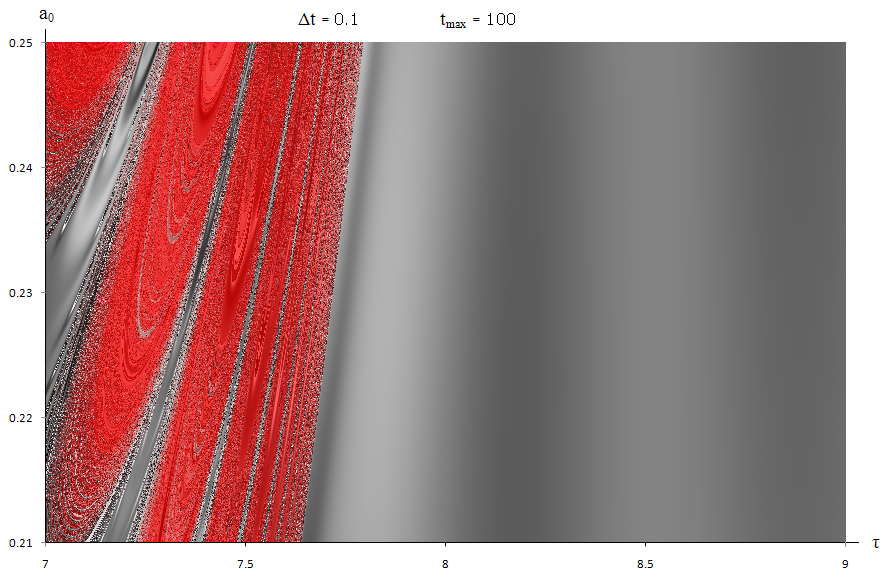Figure 6.
Values of θ for 0.21 < a0 < 0.25 and 7 < τ < 9 with tmax = 100 Red indicates θ went outside the region ( -π , π )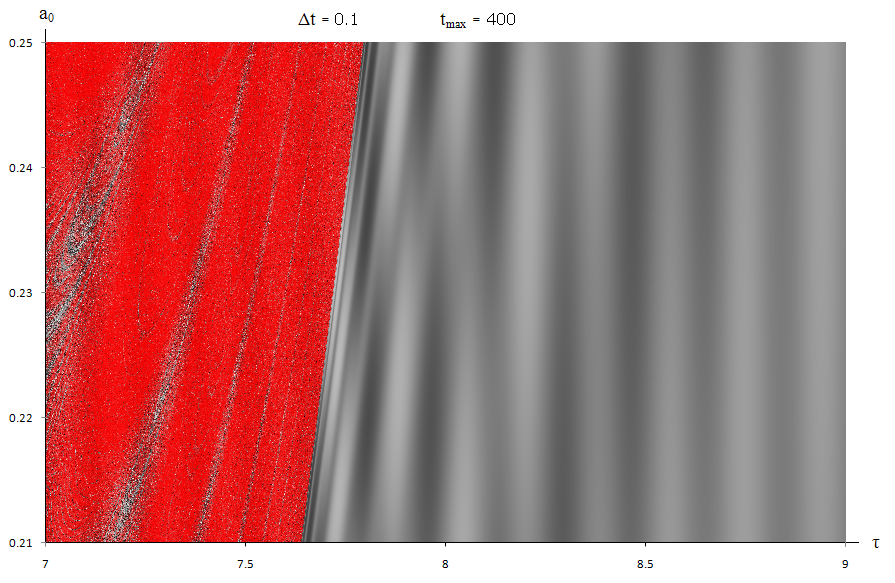Figure 7.
Values of θ for 0.21 < a0 < 0.25 and 7 < τ < 9 with tmax = 400
A deeper hue means θ spent a bigger fraction of the simulation outside the region ( -π , π )

As time evolves, the chaotic regions become clearer and spread asymptotically. The red highlight follows the same area exactly, as can be seen by comparing Figures 2&3 to Figures 6&7. Zooming out, the boundary of chaos surrounds the regular, predictable pattern in a parabola (Figure8). Outside this region the pendulum behaves like a single pendulum as the amplitude of M is too small, or the period of M is too large, to have an effect. The base of the region marks the resonant frequency of the lower pendulum (ref3).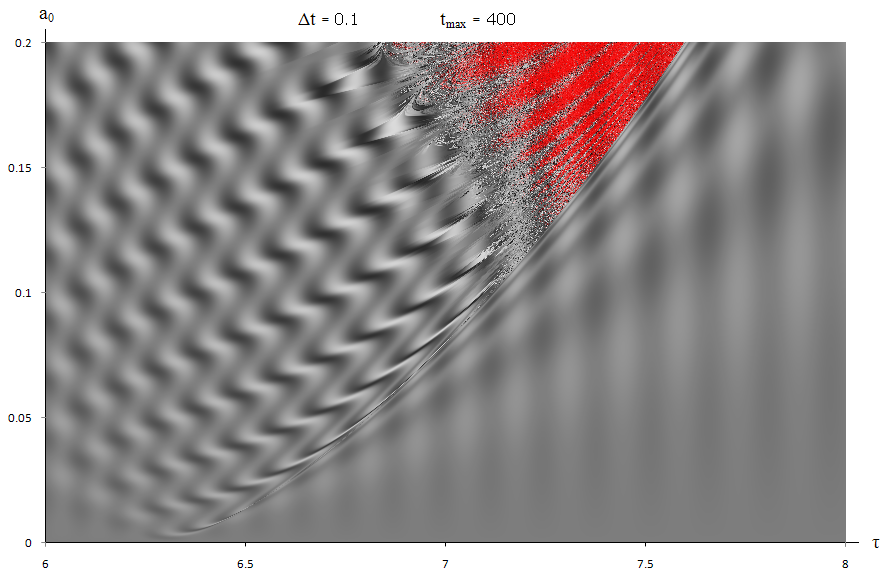Figure 8.
Values of θ for 0.0 < a0 < 0.2 and 6 < τ < 8 with tmax = 400 The chaotic region draws out a parabola over time.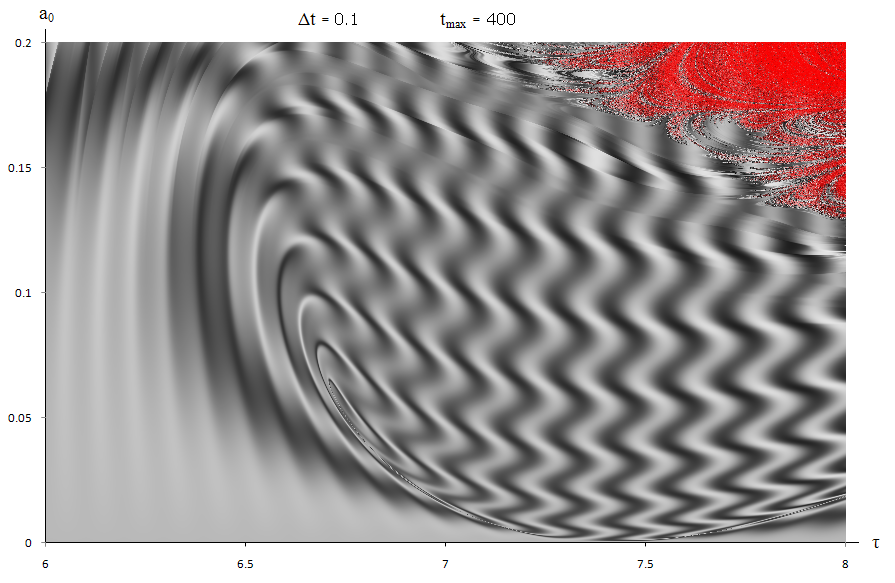Figure 9.
Same as figure 8 but with θinit=π/2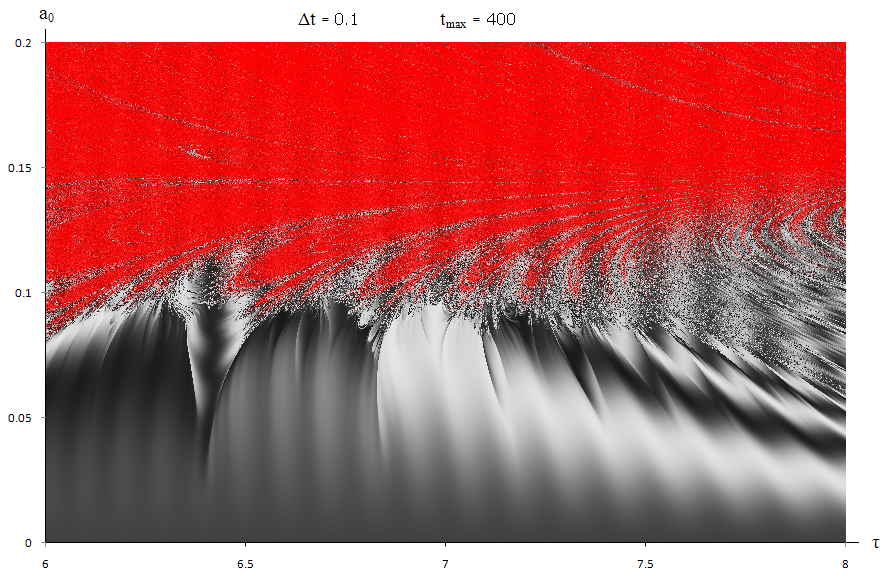Figure 10.
Same as figure 8 but with θinit=3π/4

Investigating the dependency on initial conditions, Figures 9&10 show how the same area changes when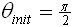and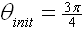(ωinit is kept constant). The chaotic region grows as the pendulum can escape the normal region much easier. At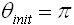the graph becomes pure chaos. Changing ωinit would produce similar (but skewed) graphs, akin to jumping ahead in the simulation.

Choosing levels of accuracy is a compromise with computing power and available time. Reducing Δt makes the regions clearer but takes longer to render. Throughout the simulations Δt=0.1, chosen after examining Figure12.

Throughout, all decimal variables were stored as double floats (8 bytes). This gives around 16 places of precision. The limitations of the program are visible when simulations are made in steps of ~10-17 (Figure11). When investigating chaos, rounding errors can have a huge effect on the output (ref2). However, the graphs in this simulation were plotted in steps of ~10-5 hence rounding errors should not have effected the results significantly.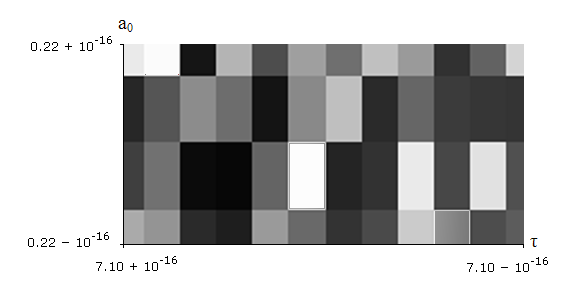Figure 11.
Extremely high resolution plots become “pixilated” as the limitations of variable accuracy become evident.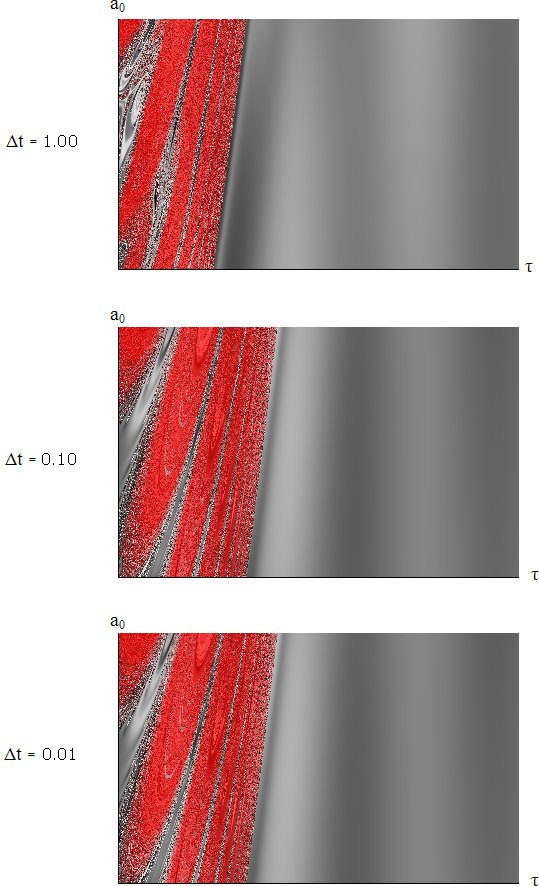Figure 12.
Three test plots of the same area for different values of Δt. The upper and centre plots show noticeable differences, whereas the lower and centre plots have negligible difference, justifying the use of Δt=0.1 as a compromise.

The model itself is only an approximation, assuming the angle of M remains small. This has shown the basic concepts of a double pendulum but a more relevant result would be achieved if mM. A plot of initial angles for both pendulums on either axis would produce a result similar to Figure13.Figure 13.
Double Pendulum plot by Jeremy S. Heyl
The colour of each pixel indicates whether either pendulum of a double pendulum flips within 10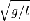(green), within 100(red), 1000(purple) or 10000(blue). Those that don't flip within 10000are plotted white. The angle that the upper pendulum makes with the vertical initially ranges from -3 at the left-hand side of the plot to +3 at the right-hand side. The angle that the lower pendulum initial makes with the vertical ranges from -3 at the top to +3 at the bottom.

References
1. David Clements, First Year Computing Laboratory, Version 2.9
2. Raymond Sneyers (1997) "Climate Chaotic Instability: Statistical Determination and Theoretical Background", Environmetrics, vol. 8, no. 5, pages 517–532.
3. Meirovitch, Leonard (1986). Elements of Vibration Analysis (2nd edition ed.). McGraw-Hill Science/Engineering/Math.